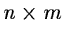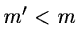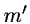Next: Cluster Analysis Up: Multivariate Analysis Methods Previous: Introduction

# Principal Components Analysis

Among the objectives of Principal Components Analysis are the following.

1.
dimensionality reduction;

2.
the determining of linear combinations of variables;

3.
feature selection: the choosing of the most useful variables;

4.
visualisation of multidimensional data;

5.
identification of underlying variables;

6.
identification of groups of objects or of outliers.

The tasks required of the analyst to carry these out are as follows:

1.
In case of a table of dimensions, each of the n rows or objects can be regarded as an m-dimensional vector. Finding a set ofprincipal axes allows the objects to be adequately characterised on a smaller number of (artificial) variables. This is advantageous as a prelude to further analysis as thedimensions may often be ignored as constituting noise; and, secondly, for storage economy (sufficient information from the initial table is now represented in a table withcolumns). Reduction of dimensionality is practicable if the firstnew axes account for approximately 75 % or more of the variance. There is no set threshold, -- the analyst must judge. The cumulative percentage of variance explained by the principal axes is consulted in order to make this choice.

2.
If the eigenvalue is zero, the variance of projections on the associated eigenvector is zero. Hence the eigenvector is reduced to a point. If this point is additionally the origin (i.e. the data is centred), then this allows linear combinations between the variables to be found. In fact, we can go a good deal further: by analysing second-order variables, defined from the given variables, quadratic dependencies can be straightforwardly sought. This means, for example, that in analysing three variables, y1, y2, and y3, we would also input the variables y12, y22, y32, y1y2, y1y3, and y2y3. If the linear combination

y1 = c1 y22 + c2 y1y2

exists, then we would find it. Similarly we could feed in the logarithms or other functions of variables.

3.
In feature selection we want to simplify the task of characterising each object by a set of attributes. Linear combinations among attributes must be found; highly correlated attributes (i.e. closely located attributes in the new space) allow some attributes to be removed from consideration; and the proximity of attributes to the new axes indicate the more relevant and important attributes.

4.
In order to provide a convenient representation of multidimensional data, planar plots are necessary. An important consideration is the adequacy of the planar representation: the percentage variance explained by the pair of axes defining the plane must be looked at here.

5.
PCA is often motivated by the search for latent variables. Often it is relatively easy to label the highest or second highest components, but it becomes increasingly difficult as less relevant axes are examined. The objects with the highest loadings or projections on the axes (i.e. those which are placed towards the extremities of the axes) are usually worth examining: the axis may be characterisable as a spectrum running from a small number of objects with high positive loadings to those with high negative loadings.

6.
A visual inspection of a planar plot indicates which objects are grouped together, thus indicating that they belong to the same family or result from the same process. Anomalous objects can also be detected, and in some cases it might be of interest to redo the analysis with these excluded because of the perturbation they introduce.Next: Cluster Analysis Up: Multivariate Analysis Methods Previous: Introduction
Petra Nass
1999-06-15